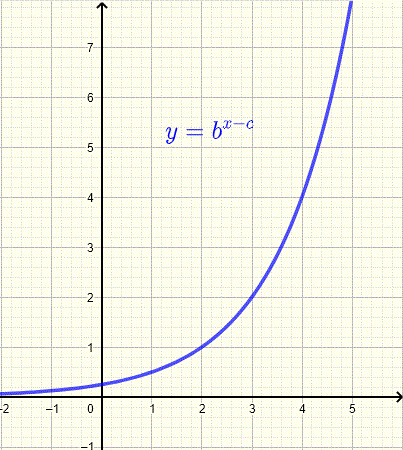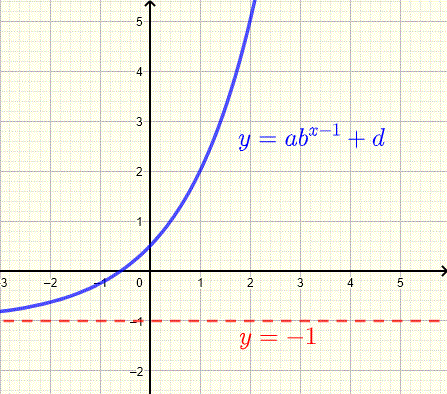# Find Exponential Function Given its Graph

## Exponential Functions

Find a possible equation of an exponential functions , given its graph, of the form $f(x) = a b^{x-c}+ d$

You may want to work through the tutorial on graphs of exponential functions to explore and study the properties of the graphs of exponential functions before you start this tutorial about finding exponential functions from their graphs.

## Examples with Detailed Solutions

Example 1
Find the exponential function of the form $y = b^x$ whose graph is shown below.Solution to Example 1
Reading the graph, we note that for $x = 1$, $y = 4$. Substitute $x$ and $y$ by their values in the equation $y = b^x$ to obtain
$b^1 = 4$
Simplify to obtain
$b = 4$
The function whose graph is shown above is given by
$y = 4^x$

Example 2
Find the exponential function of the form $y = b^{x-c}$ whose graph is shown below.Solution to Example 2
Reading the graph, we note that for $x = 2$, $y = 1$ and for $x = 3$, $y = 2$. Substitute $x$ and $y$ by their values in the equation $y = b^{x-c}$ to obtain two equations
$b^{2 - c} = 1 \; \;$ (equation 1)
and
$b^{3 - c} = 2 \; \;$ (equation 2)
Equation (1) may be written as
$b^{2 - c} = b^0$
hence
$2 - c = 0$
Which gives
$c = 2$
Substitute $c$ by $2$ in equation (2) to obtain
$b^{3 - 2} = 2$
Simplify
$b = 2$
The function whose graph is shown above is given by
$y = 2^{x - 2}$

Example 3
Find the exponential function of the form $y = - b^x + d$ whose graph is shown below.Solution to Example 3
Reading the graph, we note that for $x = 0$, $y = 0$ and for $x = 1$, $y = - 2$. Hence the two equations
$- b^0 + d = 0 \; \;$ (equation 1)
and
$- b^1 + d = - 2 \; \;$ (equation 2)
Since $b^0 = 1$, equation (1) may be written as
$- 1 + d = 0$
which gives
$d = 1$
Substitute $d$ by $1$ in equation (2) to obtain
$- b^1 + 1 = - 2$
Simplify to obtain
$b = 3$
The function whose graph is shown above is given by
$y = - 3^x + 1$

Example 4
Find the exponential function of the form $y = a \cdot b^x + d$ whose graph is shown below with a horizontal asymptote (red) given by $y = 1$.Solution to Example 4
The given graph increases and therefore the base $b$ is greater that $1$.
As $x$ decreases indefinitely ( $\lim_{x\to - \infty}$ ) , the term $a \cdot b^x$ approaches zero and $y$ approaches $d$. Therefore, the horizontal asymptote of the exponential function $y = a \cdot b^x + d$ is given by the horizontal line $y = d$, hence
$d = 1$
Reading the graph, we note that for $x = 0$, $y = 4$. Hence the equation
$a \cdot b^0 + 1 = 4$ (equation 1)
Since $b^0 = 1$, equation (1) may be written as
$a + 1 = 4$; hence
$a = 3$
Point $(1,7)$ is on the graph of the function; hence the equation
$3 \cdot b^1 + 1 = 7$
Solve for $b$ to obtain
$b = 2$
The function whose graph is shown above is given by
$y = 3 \cdot 2^x + 1$

Example 5
Find the exponential function of the form $y = a \cdot e^{x-1} + d$ whose graph is shown below with a horizontal asymptote (red) given by $y = - 2$.Solution to Example 5
The horizontal asymptote of the exponential function $y = a \cdot e^{x - 1} + d$ is given by $y = d$, hence
$d = - 2$
From the graph, we note that for $x = 1$, $y = - 4$. Hence the equation
$a \cdot e^{1 - 1} - 2 = - 4$ (equation 1)
Simplify the above to obtain the equation
$a \cdot e^0 = - 2$ (equation 1)
Since $e^0 = 1$, the above equation gives
$a = - 2$
The function whose graph is shown above is given by
$y = - 2 \cdot e^{x-1} - 2$

Example 6
Find the exponential function of the form $y = a \cdot b^{x-1} + d$ whose graph is shown below with a horizontal asymptote (red) given by $y = - 1$.Solution to Example 6
The horizontal asymptote of the exponential function $y = a \cdot b^{x-1} + d$ is given by $y = d$, hence
$d = - 1$
Reading the graph, we note that for $x = 1$, $y = 2$ and $y = 5$ for $x = 2$. Substitute $x$ and $y$ by the given values to obtain the equations
$a \cdot b^{1-1} - 1 = 2$ (equation 1)
and
$a \cdot b^{2-1} - 1 = 5$ (equation 2)
Equation (1) gives
$a b^0 = 3$
$a = 3$
Substitute $a$ by $3$ in equation (2) to obtain
$3 \cdot b^{2-1} - 1 = 5$
Solve the above equation to obtain
$b = 2$
The function whose graph is shown above along with its asymptote is given by
$y = 3 \cdot 2^{x-1} - 1$

Find the exponential function of each graph shown below. The broken line is the asymptote of the graph with the same color.1. (A): $y = e^{x-1}$
2. (B): $y = -2 \cdot 2^x - 2$
3. (C): $y = -(\dfrac{1}{2})^{x+3}+1$

## More References and Links to Exponential Functions

graphs of exponential functions
exponential functions
Exponential Functions - Interactive Tutorial.
Tutorial on Exponential Functions (1)
Tutorial on Exponential Functions (2)